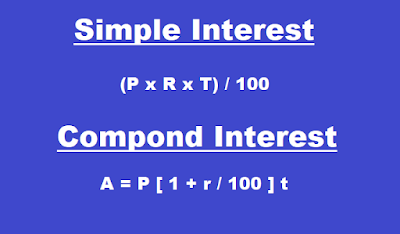# Formulae of Simple and Compound Interest

Formulae of Simple and Compound Interest - Having a problem in calculating simple and compound interest.
Here are the formulae of Simple and Compound Interest which will help you to calculate simple interest and compound interest compounded quarterly, half-yearly, monthly and yearly. Let's start with a small description of the topic which will help you to understand the formula easily.Formulae of Simple and Compound Interest | Compounded quarterly, half-yearly, monthly and yearly

## COMPOUND INTEREST

Money is said to be lent at Compound Interest (CI) when at the end of the year or fixed period the interest that has become due is not paid to the lender but is added to the sum lent, and the amount thus obtained becomes the principal for the next period. The process is repeated until the amount for the last period has been found. The difference between the original principal and the final amount is called Compound Interest (CI).

Important Formulae of Compound Interest:

Case I: When interest is compounded annually:
Amount = P [1 + r/100]t

Case II: When interest is compounded half-yearly:
Amount = P [1 + (r/2)/100]2t = P [1 + r/200]2t

Case III: When interest is compounded quarterly:
Amount = P [1 + (r/4)/100]4t  = P [1+r/400]4t

Case IV: When the rate of interest is r1%, r2% and r3% for 1st year, 2nd year and 3rd year respectively, then
Amount = P [1 + r1/100] x [1 + r2/100] x [1 + r3/100]

Case V: When interest is compounded monthly:
Amount = P [1 + (r/12)/100]12t

### SIMPLE  INTEREST

Interest is the money paid by the borrower to the lender for the use of money lent. The sum lent is called the principal. Interest is usually calculated at the rate of so many rupees for every Rs100 of the money lent for a year. This is called the rate percent per annum. Per annum means for a year.

Formulae of Simple Interest

If Principal = Rs ‘P’, Time = ‘T’ years, Rate= ‘R%’ per annum,

Simple Interest (SI) = P x R x T/100

Amount = Principal + Simple Interest

A = P + (P x R x T)/100 = P [1 + RT/100]

Also Read: Formulas and Short tricks on Percentage
Profit and Loss Important Mathematical Formulas